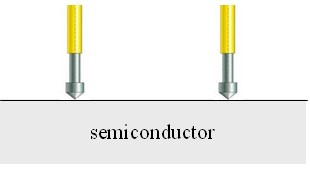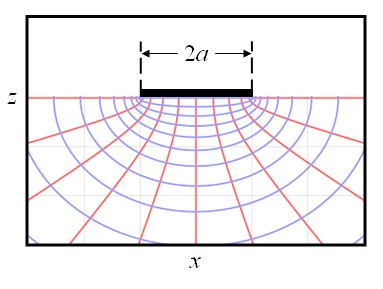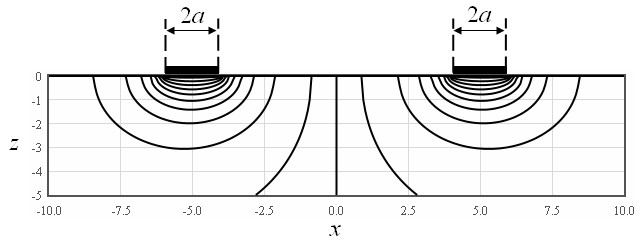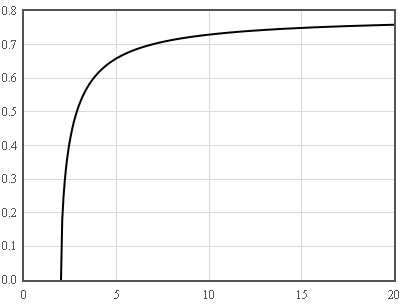Experimental Laboratory Exercises

Spreading Resistance Profiing (SRP) is a method to measure the resistivity of semiconducting samples as a function of position. Two metal needles spaced about 50 μm apart are pressed with a force of about 100 μN (10 mg) against the surface of the semiconductor and the resistance between these to needles is measured.Here we calculate the resistance that would be measured between two round metal contacts placed on a semiconducting surface assuming that there is ohmic contact between the metal and the semiconductor. If one round metal contact with a radius $a$ is placed on a semiconductor, the expressions for the potential, the electric field, and the current density are,

$\phi=\frac{\rho I}{\pi a}\tan^{-1}\left(\text{tanh}\frac{\mu}{2}\right).$

$\large \vec{E}=-\frac{\rho I \text{sech}\mu}{2\pi a^2 \sqrt{\text{sinh}^2\mu+\sin^2\nu}}\hat{\mu}.$

$\large \vec{j}=\frac{I \text{sech}\mu}{2\pi a^2 \sqrt{\text{sinh}^2\mu+\sin^2\nu}}\hat{\mu}.$

Here $\phi$ is the electrostatic potential, $\vec{E}$ is the electric field, $\vec{j}$ is the current density, $I$ is the current through the contact, and $\rho$ is the resistivity. In these formulas, the position is specificed in oblate spheroidal coordinates $(\mu,\nu,\theta)$. The derivation of these formulas can be found in the section on Maxwell contacts.The equipotentals (blue) and current density (red) for a single round contact on a semiconductor.

When two metal contacts with radii $a_1$ and $a_2$ are positioned at $x=-d/2$ and $x=d/2$ on the surface of the semiconductor, we can add the potentials from two single contacts to determine the resulting potential.

$\phi=\frac{\rho I}{\pi}\left(\frac{1}{a_1}\tan^{-1}\left(\text{tanh}\frac{\mu_1}{2}\right)-\frac{1}{a_2}\tan^{-1}\left(\text{tanh}\frac{\mu_2}{2}\right)\right).$

In the $y=0$ plane,

$\large \mu_1 =\text{cosh}^{-1}\left(\frac{\sqrt{\left(x+\frac{d}{2}+a_1\right)^2+z^2}+\sqrt{\left(x+\frac{d}{2}-a_1\right)^2+z^2}}{2a_1}\right),$

$\large \mu_2 =\text{cosh}^{-1}\left(\frac{\sqrt{\left(x-\frac{d}{2}+a_2\right)^2+z^2}+\sqrt{\left(x-\frac{d}{2}-a_2\right)^2+z^2}}{2a_2}\right).$This figure shows the equipotentials when current is passed from one electrode to the other. Most of the voltage falls near the contacts.

To calculate the voltage between the contacts, we can evaluate the potential at the surface of the semiconductor where $y=0$ and $z=0$. Along this line,

$\large \mu_1 =\text{cosh}^{-1}\left(\frac{|x+\frac{d}{2}|}{a_1}\right).$

$\large \mu_2 =\text{cosh}^{-1}\left(\frac{|x-\frac{d}{2}|}{a_2}\right).$

The voltage between the two contacts is,

$\large V=\frac{\rho I}{\pi}\left(\frac{1}{a_1}\tan^{-1}\left(\text{tanh}\left(\frac{1}{2}\text{cosh}^{-1}\frac{d-a_2}{a_1}\right)\right)+\frac{1}{a_2}\tan^{-1}\left(\text{tanh}\left(\frac{1}{2}\text{cosh}^{-1}\frac{d-a_1}{a_2}\right)\right)\right).$

The resistance between the two contacts is,

$\large R_{SRP}=\frac{V}{I}=\frac{\rho }{\pi}\left(\frac{1}{a_1}\tan^{-1}\left(\text{tanh}\left(\frac{1}{2}\text{cosh}^{-1}\frac{d-a_2}{a_1}\right)\right)+\frac{1}{a_2}\tan^{-1}\left(\text{tanh}\left(\frac{1}{2}\text{cosh}^{-1}\frac{d-a_1}{a_2}\right)\right)\right).$

The resistance is plotted below for $a_1=a_2=a$. The resistance measured in an SRP experiment is a very weak function of the distance between the electrodes as soon as that distance is a few times the radius of the contacts. For $d<2a$ the contacts overlap and the resistance is zero.

 $\large \frac{\pi a R_{SRP}}{\rho}$$\large \frac{d}{a}$